Categories

## Floating Point Woes in Three Dimensions

Sometimes, stuff in space rotates[citation needed]. In this article, I am going to explain the basic idea to simulate rotation efficiently, and a few technical hurdles I encountered while implementing it.

I have not yet got down to reinventing the wheel writing a physics engine. Besides, I don’t want to simulate physics just to keep track of the orientation of the object through time. Doing so would drastically limit how much we can time-wrap (to maybe ×10,000).

Instead, we can just assume a constant rotation (angular velocity), and deduce the orientation at any given time from the base orientation and the elapsed time. In other words, we set:

Orientation(t) = Orientation(t₀) + AngularVelocity × (t – t₀).

This approach fits very well with planets, whose angular velocity can be safely assumed to be constant.

To handle simple changes in angular velocity, we can just update t₀. So, when the ship thrusts its RCS to pivot, we set t₀ to the current time, Orientation(t₀) to the current orientation, and update the angular velocity.

Of course, the assumptions we make are not very accurate for non-spherical objects. For instance, we will not observe the Dzhanibekov effect (also known as tennis racket theorem) with this approach.

One thing remaining: how do we represent an orientation or an angular velocity? There are essentially three ways:

• Euler angles
• axis-angle
• rotation matrix
• quaternion

Let’s go over each in more detail.

Euler angles use three angles around fixed axes. They are somewhat intuitive, but are very inconvenient to work with.

(source)

You can also find the rotation’s axis to get the axis-angle representation. You’ll need three values to describe the axis, and one for the angle.

(source)

Rotation matrices can be easily combined. They also adapt well to represent translation and scaling, when using homogeneous coordinates (convenient for 3D rendering). But they need at least 9 parameters.

(source)

The theory behind quaternions is hard to grasp, but you do not need to. What matters is that you can easily combine them, extrapolate them, or interpolate between two orientations, and they only use four values.

Actually, an intuitive way to think about them is that the first components x, y and z correspond to the axis of rotation, while the w component represents the angle of the rotation: if your angle of rotation is θ, then w=cos(θ/2). We just need to keep their norm to 1, so we scale x, y and z appropriately.

(source)

So, clearly, I use quaternions wherever I can. But there is one thing to keep in mind. Quaternions (almost) uniquely represent a rotation, and a rotation by angle x is the same as the rotation by angle 2π + x around the same axis. So, if we try to store an angular velocity of 2π rad/s (one rotation per second) as a quaternion, we’ll just have a quaternion representing an angular velocity of 0 rad/s (well, 0 rotation per second).

The usual way around this is to represent angular velocity (and impulse) as axis-angle, since we can store whatever we want in the angle. But I like edge cases and floating point Eldritch horrors. So, instead, I just scale the angle down by something like K=2¹⁰⁰. We will be fine as long as the angular velocity is smaller than 7.96×10³⁰ rad/s (26 billion billion (no typo) times faster than the fastest spinning object ever).

To summarize so far, we’ll use a quaternion to represent both the rotation and the angular velocity, except that we will scale the angle of the angular velocity by some gigantic value K.

It means we will create quaternions with very small angles.

It means that the component w will be extremely close to 1 (the distance will generally be about 1 / K = 2⁻¹⁰⁰).

It means that the component w will just be equal to 1 (since even double precision floating point numbers won’t have enough precision to tell the difference). We just lost all our precision!

It means that, when retrieving the angle from w (needed when scaling by t – t₀), we will just get 0.

It means that we will multiply 0 by K.

And 0 multiplied by a finite value (even very large) is still zero.

So nothing will ever spin. Great… back to Euler angles I guess?

Not so fast! Although we cannot retrieve small angles from the w component, the information is actually present in the other ones, x, y and z!

But you just told us that the x, y and z components are just the axis of rotation! Did you lie to us? Must my dreams always come crashing done at the altar of floating point numbers. I knew I should have gone with Cthulhu.

A sensible person when confronted with floating point arithmetic

It is true! But an axis is just a direction. When these three value also store a magnitude. Remember when I told you that we had to scale x, y and z so that the norm of the quaternion stays equal to 1? Well, that’s convenient, because it means that √(x² + y² + z²) = sin(θ/2).

And sin(θ/2) is very precise around zero in floating point arithmetic!

Which means we don’t lose any precision!

After checking with Herbie and opening a merge request in GLM (the library I use for manipulating quaternions), I am able to represent angular velocity as quaternions!

Could I just have used the axis-angle representation for angular velocity? Probably? Would it have been fun? Probably not as much.

Categories

## Looking Up-Close at Orbits

In the previous post, I explained the basic idea that is used to draw orbits using line segments, and a little trick to make even very elliptical orbit look good. So, this is where we are:

But if we zoom in:

What is going on?! I thought we had taken care of spreading the line segments to hide the angle as much as possible!

A distraught hypothetical reader

Well, true. But we did it assuming we were looking straight down at the ellipse. Here, perspective comes into play. Although it conserves lines, perspective has the nasty habits of effecting angles. Here, it magnifies the angles between segments around the point where the orbits appear to bend towards the left. On the contrary, the segments on the left part look pretty much aligned.

But it’s okay. We only need to add a few more points close to the camera to compensate for this. It does mean that they will move as the object follows its orbit.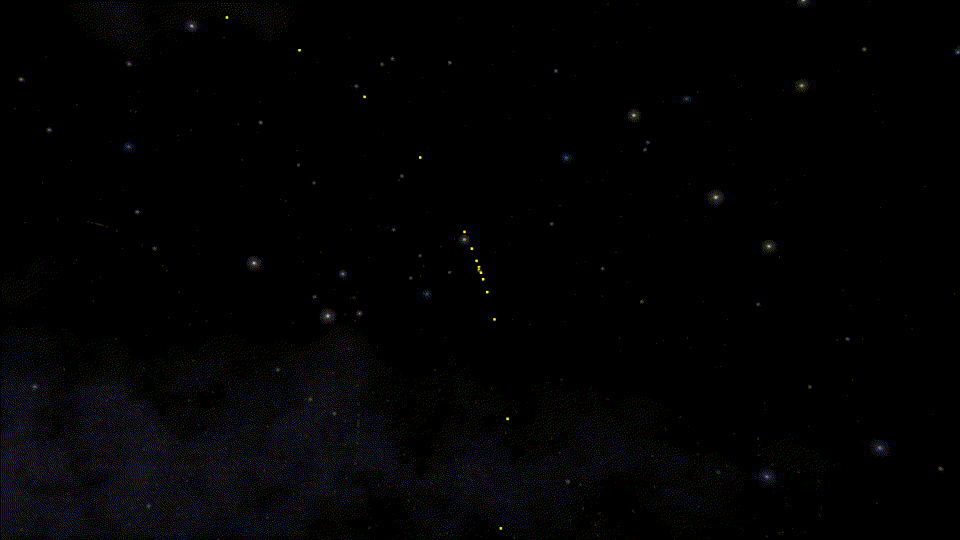Here, we can clearly see the additional points moving alongside the focused object on the orbit. When we zoom in, they make sure we don’t see any unsightly corner.

But wait, there is more!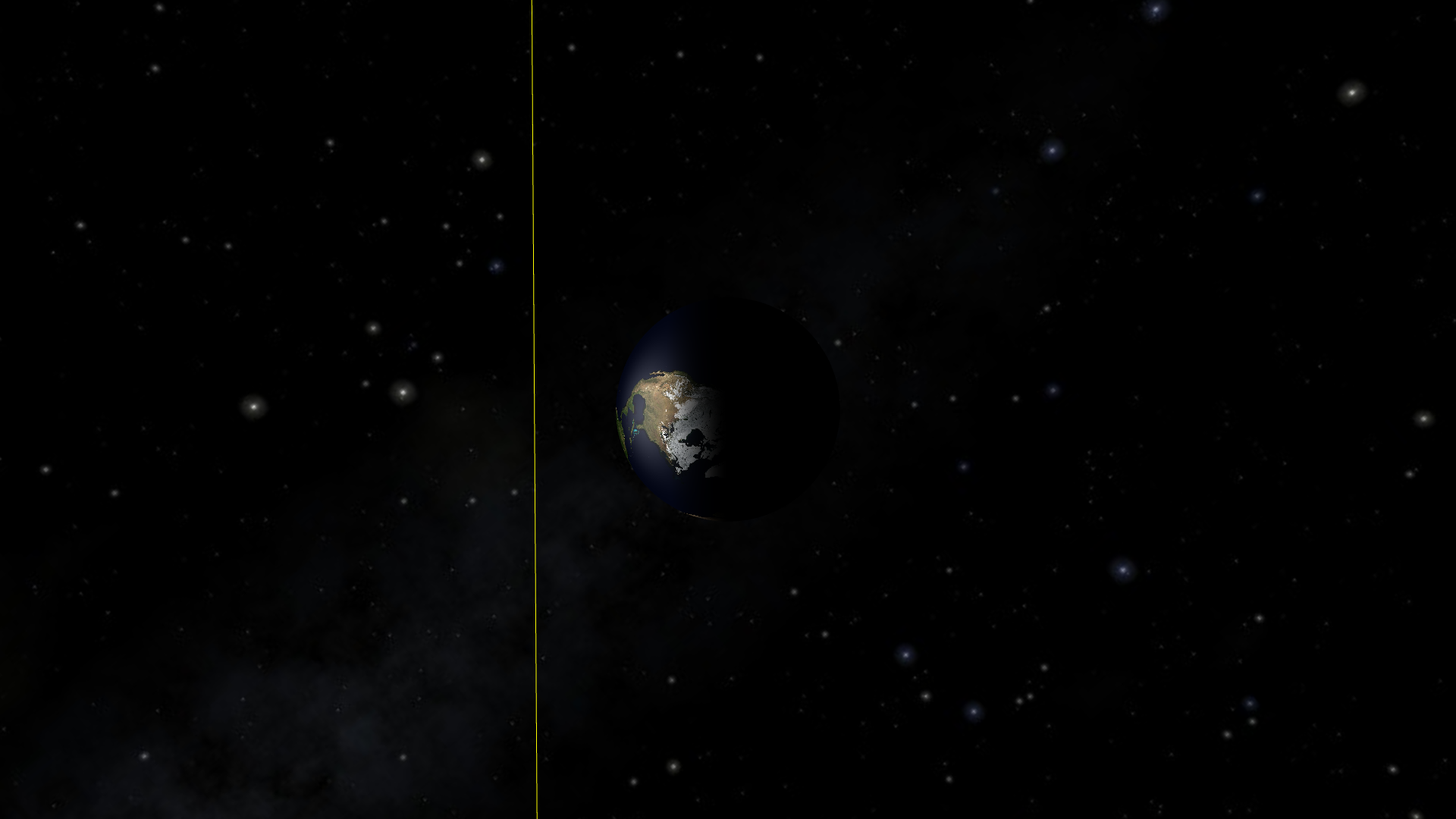The Earth not being on its own orbit might be a bit of a problem

This is not the path of the Earth-Moon barycenter, which is the surface of the Earth anyway. The line really should pass through the center of the Earth.

So, what is going on? We do have a nice smooth-looking orbit, but it is still an approximation. It just so happens that we have looked at a time when the Earth was in the middle of a line segment. In the diagram below, you can imagine that the line segment is BX and the Earth is betwen the two.

This becomes more apparent as we speed things up: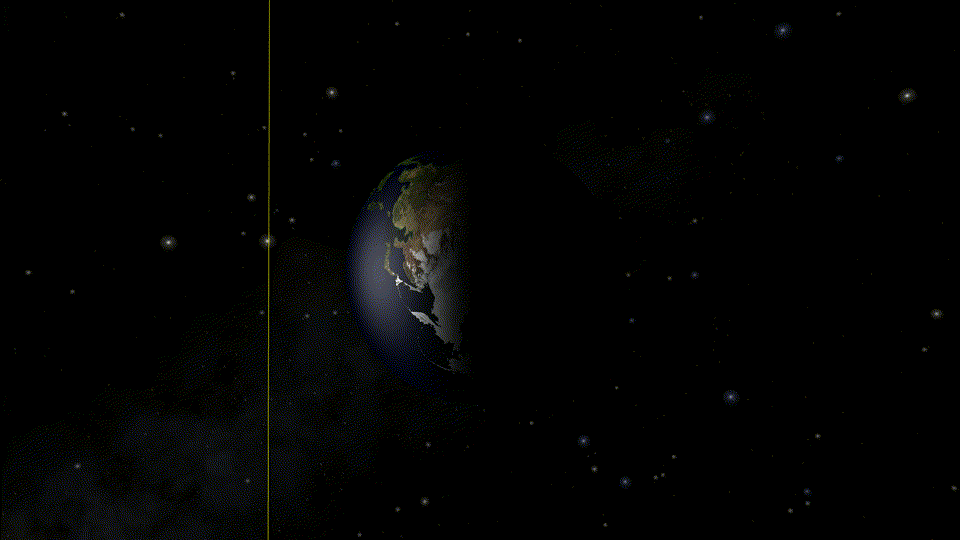The Earth follows the actual path of the ellipse while the orbit is just a bunch of line segments. The points between line segments are in the right position, which corresponds to the times when the orbit actually appear to go through Earth’s center.

To avoid this, we just need to ensure that at least one point of our orbit is always in the middle of the Earth. Actually, we can just make it so that it is one of the additional points from earlier!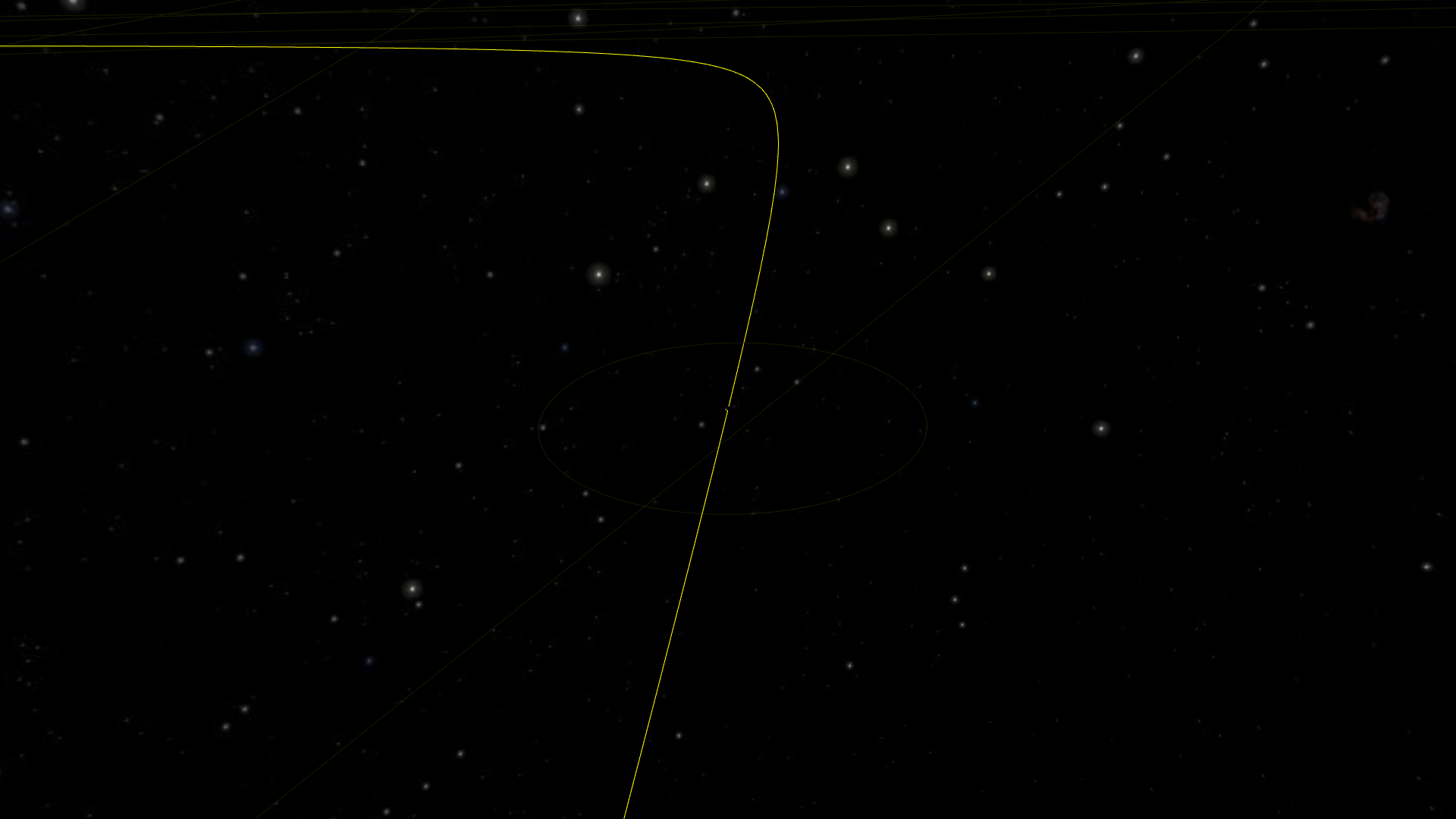Now everything looks smooth and the Earth is always on its orbit!

Actually, that was the easy part. Now let’s zoom a bit more for the fun one: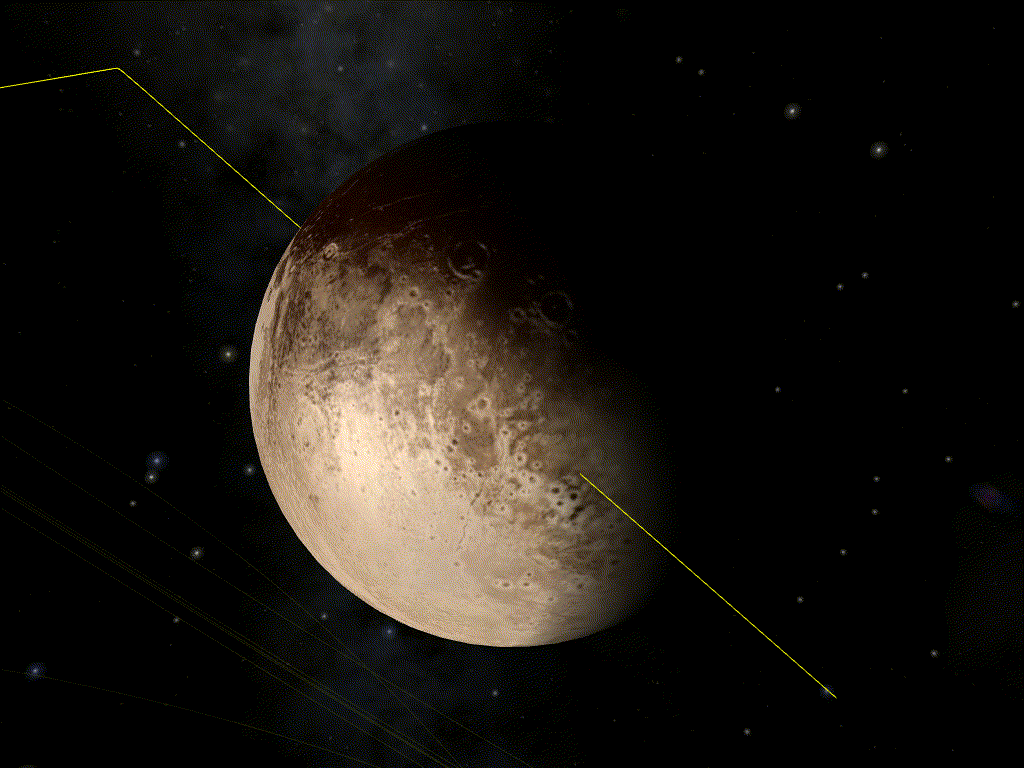Showing Pluto here, as the effect is more visible. But we cannot ignore it just because it’s not a planet anymore! And the effect is even more visible on smaller objects such as spaceships, because we can zoom closer to the orbit.

To understand what is going on, we need to dive into how we actually calculate the positions of the points of our orbit. Digital computers only know about discrete values (that’s what the “digital” mean). The basic unit is the “bit” (0 or 1) and, by using several bits, we can count natural integers fairly easily (0, 1, 10, 11, 100, 101, 110, 111, 1000). Add a sign bit, and we’ve got relative integers. We’re not going to be able to represent arbitrary real numbers short of using symbolic computation, but we can at least get something good enough by using decimals. The idea is that we would use two relative numbers: one for the number’s digit, and one to tell where the decimal point should be. And that’s exactly what computers do. Well almost.

Technically, computers use binary instead of base ten, but the principle is the same. If we really want to use base 10, we can, albeit it’s slower:

But the important point to keep in mind when working with non-integer values with computers is that they are (usually) only approximations of the number we want.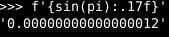For instance, the approximation of π used by Python is slightly smaller than the actual value of π. So, if we compute the sine of this approximation, we get something slightly bigger than zero.

We can get a good idea of the approximation. There are two usual formats used to represent non-integer: binary32 and binary64, more commonly known as float and double respectively (although Python’s float is binary64). The relative error of a binary32 is roughly 2⁻²⁴ and the relative error of a binary64 is roughly 2⁻⁵³.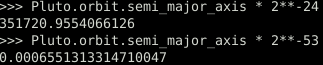The error in binary32 when dealing with values around the radius of Pluto’s orbit is of 352 km (Pluto’s radius is 1,188 km). With binary64, it’s less than a millimeter.

Rendering to a screen which is no more than 8,000 pixels a size should not require more than binary32. So this is what is normally used for rendering. Using binary64 here would degrade performance a lot. But it does mean that we are getting imprecision when drawing things naively.

Let’s say the Sun is the center of our system of coordinates, and we use SI units. Then, the Pluto is 5.9 Tm from the Sun. For rendering, we are going to compute the positions of every object relative to the Sun, compute the position of the camera (our point of view) relative to the Sun, and subtract the second from the first to find the position of the objects relatively to the camera.

Since this is naturally done during the rendering in the GPU shaders, we use binary32. The error from doing computations in binary32 is roughly 352 km in this case. So, let’s start from the camera position; we are at a distance of 0 (exactly). Then, let’s move to the Sun; we are at a distance of 5.9 Tm±352km (relatively precise). And let’s move back to Pluto; we are at distance of (5.9 Tm±352km) – (5.9 Tm±352km) = ±352 km (not very precise). This is called loss of significance.

We really want to avoid doing such a computation in binary32. We must either switch to using binary64 in the rendering, or avoid doing this computation in the shaders entirely. We really want to avoid doing the first one, since it can slow down the rendering significantly, and not all GPUs even support it.

How do we avoid doing this computation in the shaders then? The most intuitive approach seems to switch the system of coordinate to take Pluto as center. But this has issues too, since we still have the exact same imprecision when switch from one coordinate to the other. The solution I opted for is actually simple: I do the imprecise computation using binary64 outside the shaders, which lets me have millimeter precision around Pluto. It can be seen as switching coordinates, but only for part of the rendering.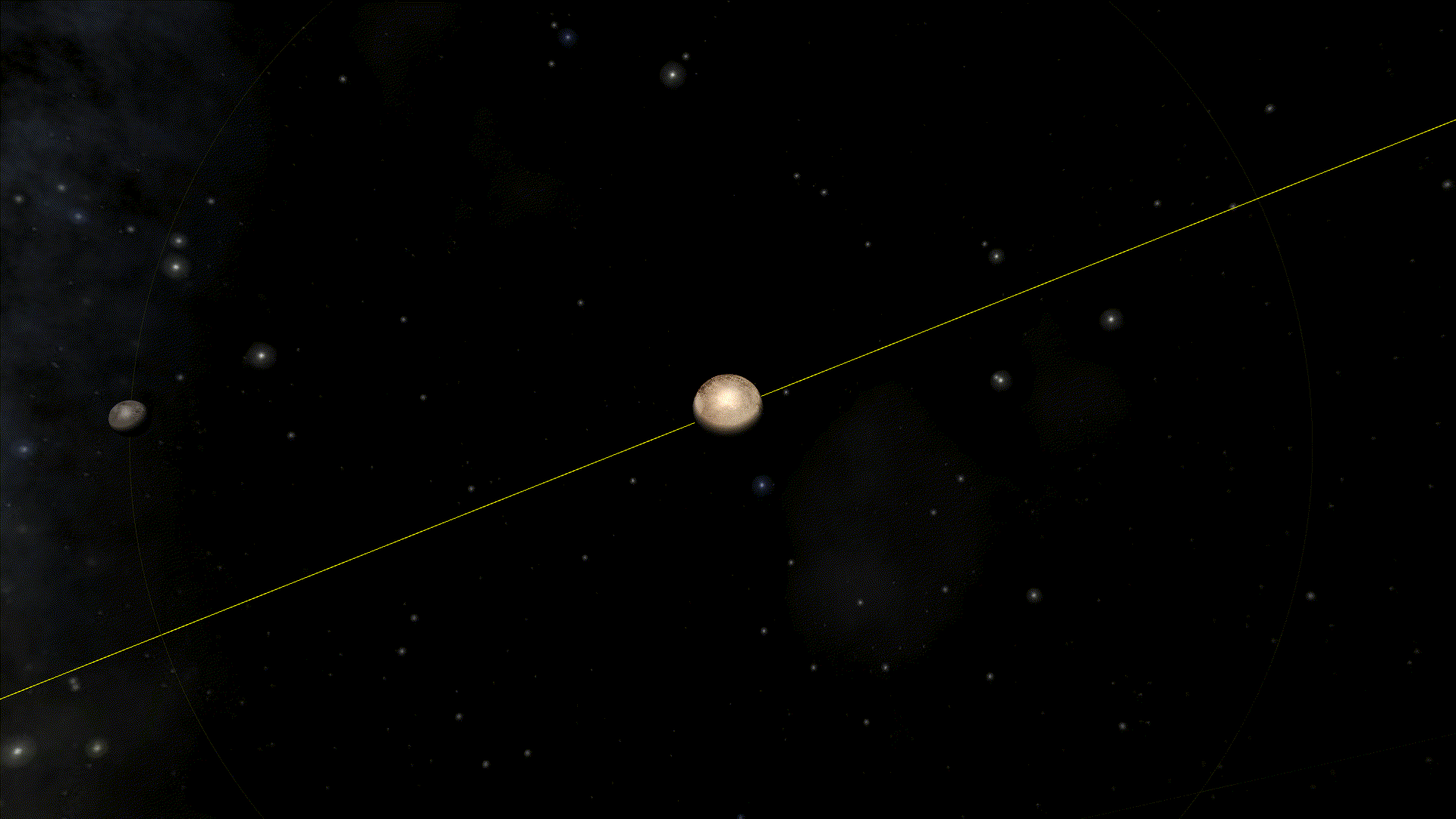The loss of significance is still here. But it’s now only 1 mm.

Note: I use the same technique when drawing celestial bodies. This is actually why the object being observed is well positioned at the center of the screen and do not move erratically (unlike in the example below).

This is not all roses however. For instance, if we look at Pluto from the Earth.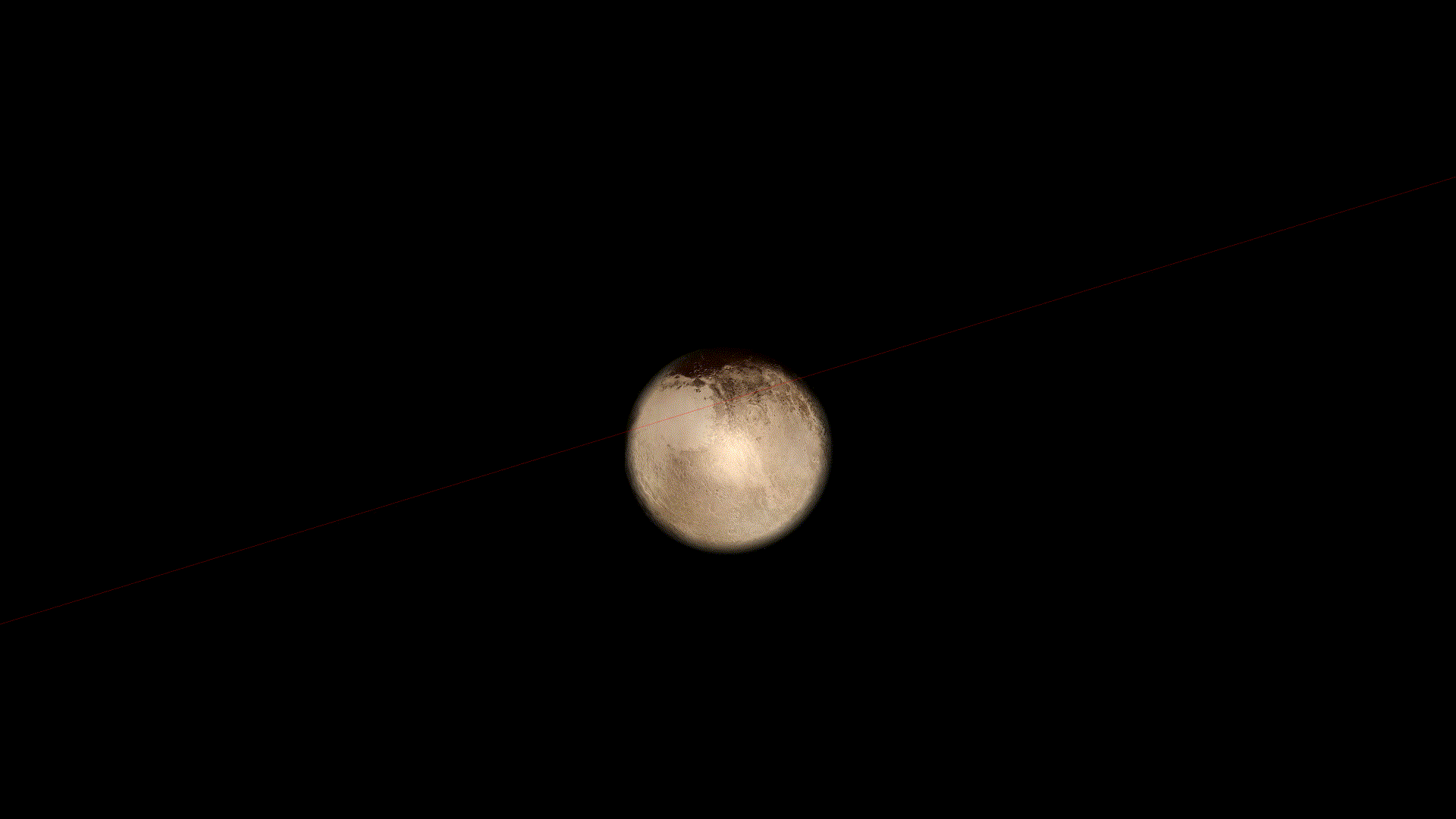This requires a FOV of 36 hundreth of an arcsecond. Current telescope are far from being able to do this, but a software simulation should.

Since the camera is positionned at the center of the Earth, the distance to Pluto is of the order of 6 Tm. With this level of zoom however, we can distinctly see the imprecision of roughly 350 km, causing the dwarf planet to not be perfectly centered in the screen.

Changing the system of coordinates is not quite enough, since the camera is not close to the observed object. So I will need ot find another workaround.

So, seriously, don’t let the floating point bugs bite.